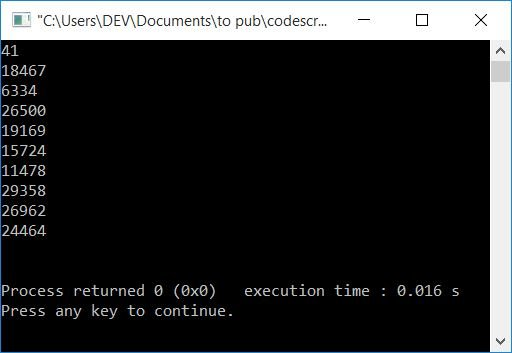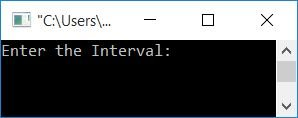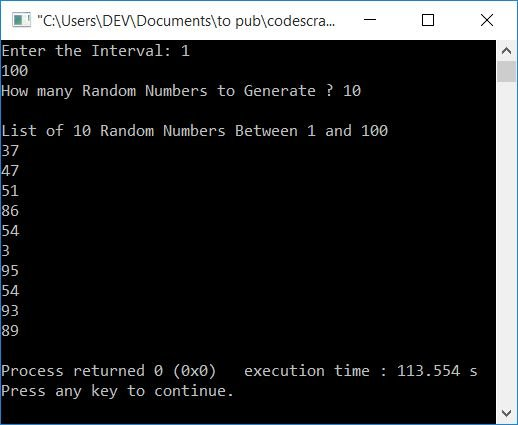# C++ program to generate random numbers

This article will teach you how to generate and print random numbers using the C++ programming language. Here is the list of programs that generates random numbers:

### How to Generate Random Numbers

To generate a random number, use the rand() function. This function is defined in the stdlib.h header file.

## Generate 10 random numbers in C++

This program generates and prints 10 random numbers using a for loop and the rand() function.

```#include<iostream>
#include<stdlib.h>
using namespace std;
int main()
{
int i;
for(i=0; i<10; i++)
cout<<rand()<<endl;
cout<<endl;
return 0;
}```

This program was built and runs under the Code::Blocks IDE. Here is its sample output:Note: If you re-run the above program, then it'll produce the same 10 random numbers as previously. So to solve this problem and generate random numbers without repetition, we've got another program for you.

## Generate random numbers with no repetition in C++

This is the program that generates random numbers without repetition. Here, we've used the srand() (seed random) function to set the initial point for generating random numbers using the time() function.

Note: The time() function is used to set the initial point for the srand() function. Here we've used the time() function because it is the thing that is continuously changing its value.

The function time() is defined in time.h header file. So don't forget to include this header file before executing the program given below. The rest of the program is identical to the previous one.

```#include<iostream>
#include<stdlib.h>
#include<time.h>
using namespace std;
int main()
{
int i;
srand(time(0));
for(i=0; i<10; i++)
cout<<rand()<<endl;
cout<<endl;
return 0;
}```

This program produces the same output as the previous program. The only difference is that every time we execute this program, we'll get random numbers without repetition. Or we'll get different random numbers.

## In C++, produce random numbers in the given range

This is the last program that generates random numbers in a given range. The user must supply the range at runtime.

That is, this program asks the user to enter the interval first. For example, if the user enters the interval as 5  and 20, then the program further asks for the amount, say 10, to generate 10 random numbers between 5 and 20. Let's have a look at the program.

```#include<iostream>
#include<stdlib.h>
#include<time.h>
using namespace std;
int main()
{
int i, rnum, amount, first, last;
cout<<"Enter the Interval: ";
cin>>first>>last;
cout<<"How many Random Numbers to Generate ? ";
cin>>amount;
cout<<"\nList of "<<amount<<" Random Numbers Between "<<first<<" and "<<last;
srand(time(0));
for(i=0; i<amount; i++)
{
rnum = rand()%(last+1-first)+first;
cout<<endl<<rnum;
}
cout<<endl;
return 0;
}```

Here is its sample run. This is the program's initial output:Now enter the interval, say 1 to 100, and then enter the amount, say 10, to generate 10 random numbers between 1 and 100. That is, after supplying these inputs, the program will print 10 random numbers, where all random numbers must be greater than or equal to 1 and less than or equal to 100:#### The same program in different languages

C++ Quiz

« Previous Program Next Program »